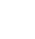# Hiding and Unhiding data in Excel

When you are dealing with worksheets with a user-function – ie a user needs to complete a questionnaire or needs to add details to specific parts, to make it easier to use and have a professional appearance it may be useful to dynamically hide or unhide rows and sections of data that become applicable during the process of adding the information and making choices.

If a user answers ‘Yes’ to a particular question, this may need to trigger only a small part of data further down the worksheet that has become applicable. Using the Hide and unhide functions in VBA can help here.

In the example image below you can see that a small section of the worksheet is actually hidden – The marketing section. This is because in this particular project, where the user selects no, the author wanted that section to be hidden. Where they have selected yes, the sections are visible.Applying this type of VBA to a project is slightly different but just as simple. Instead of adding the code to a module in the VB Editor (ALT+F11) we right click the tab and add code directly to that sheet. We can double click the applicable sheet in the VB Editor for the same outcome though.

As we are looking to hide data away from the user, we start by hiding absolutely everything that might need to be hidden, and leave it to the user to make their choices and unhide as they progress. This example works on the ‘Worksheet_Activate’ trigger – ie whenever the worksheet is opened by the user. It also means it takes into account the previous choices made and stored on the workbook.

``` Private Sub Worksheet_Activate() Application.ScreenUpdating = False Worksheets("Section B").Range("A97:A233").EntireRow.Hidden = True```

``` If ActiveSheet.Range("F63") = "Yes" Then ActiveSheet.Range("A82:A98").EntireRow.Hidden = False If ActiveSheet.Range("F63") = "Yes, Mostly" Then ActiveSheet.Range("A82:A98").EntireRow.Hidden = False If ActiveSheet.Range("F63") = "Yes, partly" Then ActiveSheet.Range("A82:A98").EntireRow.Hidden = True If ActiveSheet.Range("F63") = "No" Then ActiveSheet.Range("A82:A98").EntireRow.Hidden = True If ActiveSheet.Range("F65") = "Yes" Then ActiveSheet.Range("A99:A115").EntireRow.Hidden = False If ActiveSheet.Range("F65") = "Yes, Mostly" Then ActiveSheet.Range("A99:A115").EntireRow.Hidden = False If ActiveSheet.Range("F65") = "Yes, partly" Then ActiveSheet.Range("A99:A115").EntireRow.Hidden = True If ActiveSheet.Range("F65") = "No" Then ActiveSheet.Range("A99:A115").EntireRow.Hidden = True ```

```If ActiveSheet.Range("F68") = "Yes" Then ActiveSheet.Range("A116:A133").EntireRow.Hidden = False If ActiveSheet.Range("F68") = "Yes, Mostly" Then ActiveSheet.Range("A116:A133").EntireRow.Hidden = False If ActiveSheet.Range("F68") = "Yes, partly" Then ActiveSheet.Range("A116:A133").EntireRow.Hidden = True If ActiveSheet.Range("F68") = "No" Then ActiveSheet.Range("A116:A133").EntireRow.Hidden = True End Sub ```

Now the code above doesn’t actually dynamically change the hidden and visible data. It will work if you flip between sheets but if you want it to work dynamically, you will need to apply the same code to the ‘Worksheet_Change’ module as below:

``` Private Sub Worksheet_Change(ByVal Target As Range) Application.ScreenUpdating = False```

``` If ActiveSheet.Range("F63") = "Yes" Then ActiveSheet.Range("A82:A98").EntireRow.Hidden = False If ActiveSheet.Range("F63") = "Yes, Mostly" Then ActiveSheet.Range("A82:A98").EntireRow.Hidden = False If ActiveSheet.Range("F63") = "Yes, partly" Then ActiveSheet.Range("A82:A98").EntireRow.Hidden = True If ActiveSheet.Range("F63") = "No" Then ActiveSheet.Range("A82:A98").EntireRow.Hidden = True If ActiveSheet.Range("F65") = "Yes" Then ActiveSheet.Range("A99:A115").EntireRow.Hidden = False If ActiveSheet.Range("F65") = "Yes, Mostly" Then ActiveSheet.Range("A99:A115").EntireRow.Hidden = False If ActiveSheet.Range("F65") = "Yes, partly" Then ActiveSheet.Range("A99:A115").EntireRow.Hidden = True If ActiveSheet.Range("F65") = "No" Then ActiveSheet.Range("A99:A115").EntireRow.Hidden = True ```

```If ActiveSheet.Range("F68") = "Yes" Then ActiveSheet.Range("A116:A133").EntireRow.Hidden = False If ActiveSheet.Range("F68") = "Yes, Mostly" Then ActiveSheet.Range("A116:A133").EntireRow.Hidden = False If ActiveSheet.Range("F68") = "Yes, partly" Then ActiveSheet.Range("A116:A133").EntireRow.Hidden = True If ActiveSheet.Range("F68") = "No" Then ActiveSheet.Range("A116:A133").EntireRow.Hidden = True End Sub ```

There are lots of other situations where you may need to hide/unhide data and this example shows the basic principal of how it works. Be careful though if you are dealing with numbers to hide/unhide rows of data as you will need to use the ‘Worksheet_Calculate’ functions and screen flicker may become an issue.

### One thought on “Hiding and Unhiding data in Excel”

1.Alex says:

Hi I’m having an issue with intermittent screenflashing on the worksheet when I’m using this code. Any ideas what I could be doing wrong at all ?